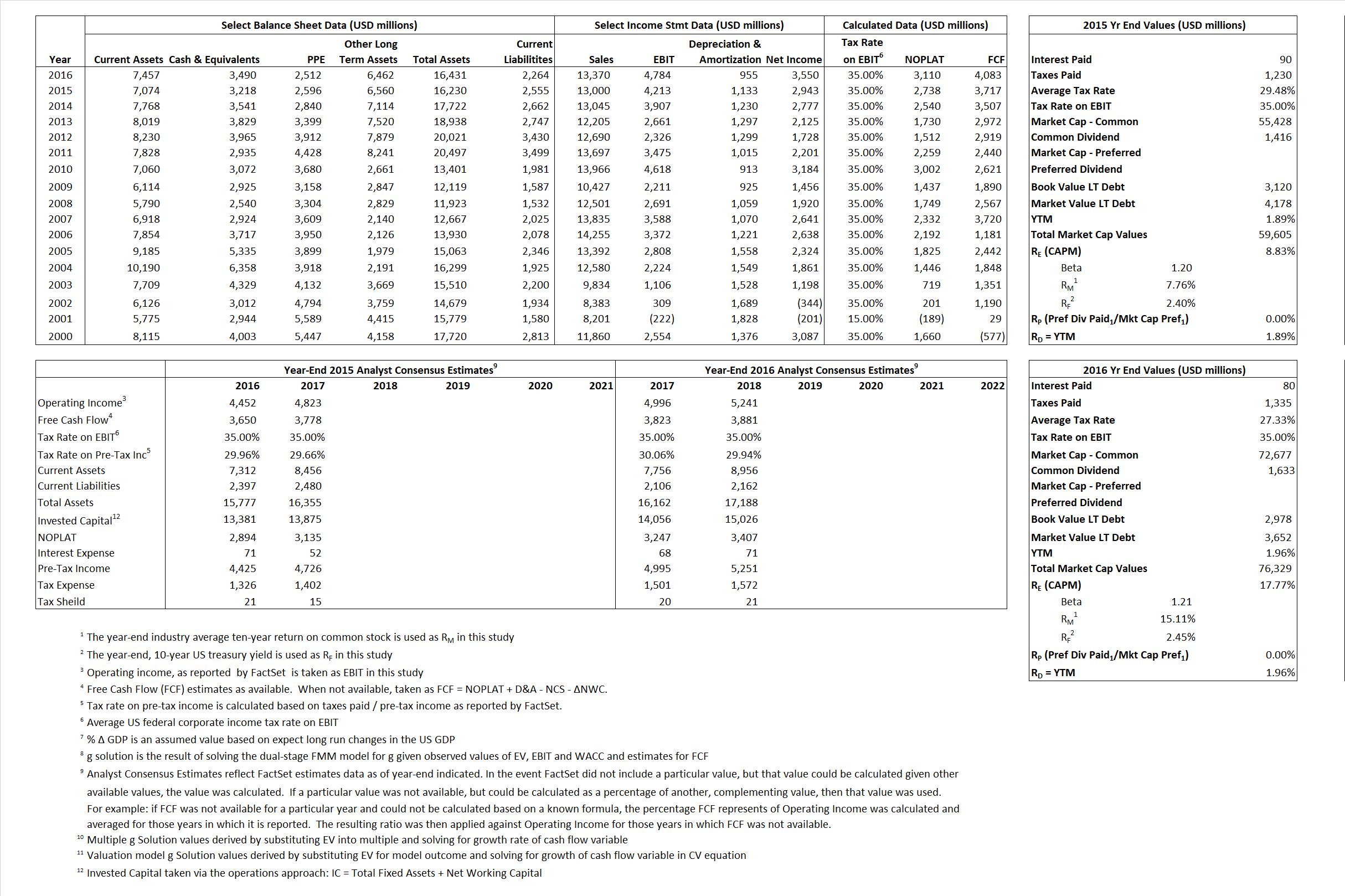# Texas Instruments

## Analyst Listing

The following analysts provide coverage for the subject firm as of May 2016:

 Broker Analyst Analyst Email BMO Capital Markets Ambrish Srivastava ambrish.srivastava@bmo.com RBC Capital Markets Amit Daryanani amit.daryanani@rbccm.com Wedbush Securities Betsy Van Hees betsy.vanhees@wedbush.com Evercore ISI C.J. Muse cj.muse@evercoreisi.com Drexel Hamilton Cody Acree cacree@drexelhamilton.com B Riley & Co Craig A. Ellis cellis@brileyco.com Wells Fargo Securities David Wong david.m.wong@wellsfargo.com CRT Capital Group Douglas Freedman dfreedman@sterneageecrt.com Raymond James J. Steven Smigie steve.smigie@raymondjames.com Credit Suisse John Pitzer john.pitzer@credit-suisse.com Jefferies Mark Lipacis mlipacis@jefferies.com Pacific Crest Securities-KBCM Michael McConnell mmcconnell@pacific-crest.com Oppenheimer Rick Schafer rick.schafer@opco.com Nomura Research Romit Shah romit.shah@nomura.com Deutsche Bank Research Ross Seymore ross.seymore@db.com Bernstein Research Stacy A. Rasgon stacy.rasgon@bernstein.com Cowen & Company Timothy Arcuri timothy.arcuri@cowen.com Stifel Nicolaus Tore Svanberg tsvanberg@stifel.com Mizuho Securities USA Vijay Rakesh vijay.rakesh@us.mizuho-sc.com SunTrust Robinson Humphrey William Stein william.stein@suntrust.com Daiwa Securities Co. Ltd. Yoko Yamada yoko.yamada@us.daiwacm.com

## Primary Input Data## Derived Input Data

### Equational Form

Net Operating Profit Less Adjusted Taxes NOPLAT  2,738 3,110$NOPLAT\, =\, EBIT\, x\, (1 \,-\, Avg \,\,Tax\,\, Rate\,\, on\,\, EBIT)$
Free Cash Flow FCF 3,717 4,083$FCF\,=NOPLAT\,+\,Non-Cash\,Expenses-\Delta NWC\,-\,NCS$
Tax Shield TS 27 22$TS\,=\,Interest\,\,Paid\,\,x\,\, Avg \,\,Tax\,\,Rate\,\, on\,\, Pre-Tax\,\, Income$
Invested Capital IC 13,675 14,167$IC\,=\,Fixed\,\,Operating\,\,Assets\,\,+\,\,Net\,\, Working\,\, Capital$
Return on Invested Capital ROIC 20.03% 21.95%$ROIC\,=\,\frac { NOPLAT }{ IC }$
Net Investment NetInv (252) 1,447$NetInv\,=\,{ {IC}_{1}}-{{IC}_{0}}+Depreciation$
Investment Rate IR -9.20% 46.53%$IR\,=\,\frac {NetInv}{NOPLAT}$
Weighted Average Cost of Capital
WACCMarket 8.31% 16.99%$WACC\,=\,\frac { E }{ V } { R }_{ E }\,+\,\frac { P }{ V } { R }_{ P }\,+\,\frac { D }{ V } { R }_{ D }\left( 1- Avg\,\, Tax\,\,Rate\,\,on\,\,Pre-Tax\,\,Income \right)$
WACCBook  10.21%  9.95%
Enterprise value
EVMarket 56,387 72,839$EV\,=\,Market\,\,Cap\,\,Equity\,+\,\,Long\,\,Term\,\,Debt\,-\,Cash$
EVBook   55,058  72,165
Long-Run Growth
g = IR x ROIC
-1.84%  10.21% Long-run growth rates of the income variable are used in the Continuing Value portion of the valuation models.
g = %$\Delta$ GDP   2.50%   2.50%
Margin from Operations M  32.41%  35.78%$M\,\,=\,\,\frac{EBIT}{SALES}$
Depreciation/Amortization Rate D  21.19%  16.64%$D\,\,=\,\,\frac{D+A}{EBITDA}$

## Valuation Multiple Outcomes

The outcomes presented in this study are the result of original input data, derived data, and synthesized inputs.

### model g solution

12/31/2015 12/31/2016 12/31/2015 12/31/2016 12/31/2015 12/31/2016

EV/SALES$\frac {EV}{Sales} \,= \,\frac{ROIC\, -\, g}{ROIC\,(WACC\,-\,g)}\,(1\,-\,T)\,(M)$

4.34  5.45  4.55%  15.79%  4.72% 16.16%

EV/EBITDA$\frac {EV}{EBITDA} \,= \,\frac{ROIC\, -\, g}{ROIC\,(WACC\,-\,g)}\,(1\,-\,T)\,(1\,-\,D)$

10.55 12.69 4.55% 15.79% 4.72% 16.16%

EV/NOPLAT$\frac {EV}{NOPLAT} \,= \,\frac{ROIC\, -\, g}{ROIC\,(WACC\,-\,g)}$

20.59 23.42 4.55% 15.79% 4.72% 16.16%

EV/FCFOPS$\frac {EV}{FCF_{OPS}} \,= \,\frac{ROIC\, -\, g}{ROIC\,(WACC\,-\,g)}\,(1\,-\,T)$

15.17 17.84 4.55% 15.79% 4.72% 16.16%

EV/EBIT$\frac {EV}{EBIT} \,= \,\frac{ROIC\, -\, g}{ROIC\,(WACC\,-\,g)}\,(1\,-\,T)$

13.38 15.23 4.55% 15.79% 4.72% 16.16%

EV/IC$\frac {EV}{IC} \,= \,\frac{ROIC\, -\, g}{WACC\,-\,g}$

4.12 5.14 4.55% 15.79% 4.72% 16.16%